# Examples for secondary school students

#### Number of problems found: 2186

• Cars plates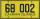How many different licence plates can country have, given that they use 3 letters followed by 3 digits?
• PrizeHow many ways can be rewarded 9 participants with the first, second, and third prizes in a sports competition?
• Bits, bytes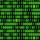Calculate how many different numbers can be encoded in 16-bit binary word?
• Harmonic HM exampleHarmonic means of 4 and 8.On the menu are 12 kinds of meal. How many ways can we choose four different meals into the daily menu?
• Vectors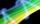For vector w is true: w = 2u-5v. Determine coordinates of vector w if u=(3, -1), v=(12, -10)
• AS - sequence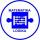What are the first ten members of the sequence if a11=22, d=2.
• CardsThe player gets 8 cards of 32. What is the probability that it gets a) all 4 aces b) at least 1 ace
• ReciprocalCalculate reciprocal of z=0.8-1.8i:
• Difference AP 4Calculate the difference of the AP if a1 = 0.5, a2 + a3 = -1.1
• GP - 8 itemsDetermine the first eight members of a geometric progression if a9=512, q=2
• Log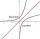Calculate value of expression log |3 +7i +5i2| .
• Sequence 3Write the first 5 members of an arithmetic sequence: a4=-35, a11=-105.
• SequenceWrite the first 7 members of an arithmetic sequence: a1=-3, d=6.
• Intersect and conjuctionLet U={1,2,3,4,5,6} A={1,3,5} B={2,4,6} C={3,6} Find the following. 1. )AUB 2. )A'UB'
• One threeWe throw two dice. What is the probability that max one three falls?
• Sequence 11What is the nth term of this sequence 1, 1/2, 1/3, 1/4, 1/5?
• Olympics metalsIn how many ways can be win six athletes medal positions in the Olympics? Metal color matters.
• GasholderThe gasholder has spherical shape with a diameter 23 m. How many m3 can hold in?
• WavelengthCalculate the wavelength of the tone frequency 11 kHz if the sound travels at speeds of 343 m/s.

Do you have an interesting mathematical word problem that you can't solve it? Submit a math problem, and we can try to solve it.

We will send a solution to your e-mail address. Solved examples are also published here. Please enter the e-mail correctly and check whether you don't have a full mailbox.

Please do not submit problems from current active competitions such as Mathematical Olympiad, correspondence seminars etc...